# Designing Kalman Filters¶

In :
from __future__ import division, print_function
%matplotlib inline

In :
#format the book
import book_format
book_format.set_style()

Out:

## Introduction¶

In the last chapter we worked with 'textbook' problems. These are problems that are easy to state, program in a few lines of code, and teach. Real world problems are rarely this simple. In this chapter we will work with more realistic examples, and learn how to evaluate filter performance.

We will begin by tracking a robot in a 2D space, such as a field or warehouse. We will start with a simple noisy sensor that outputs noisy $(x,y)$ coordinates which we will need to filter to generate a 2D track. Once we have mastered this concept, we will extend the problem significantly with more sensors and then adding control inputs.

We will then move to a nonlinear problem. The world is nonlinear, but the Kalman filter is linear. Sometimes you can get away with using it for mildly nonlinear problems, sometimes you can't. I'll show you examples of both. This will set the stage for the remainder of the book, where we learn techniques for nonlinear problems.

## Tracking a Robot¶

This first attempt at tracking a robot will closely resemble the 1D dog tracking problem of previous chapters. Instead of a sensor that outputs position in a hallway, we now have a sensor that supplies a noisy measurement of position in a 2D space. At each time $t$ it will provide an $(x,y)$ coordinate pair of the noisy measurement of the sensor's position in the field.

Implementation of code to interact with real sensors is beyond the scope of this book, so as before we will program simple simulations of the sensors. We will develop several of these sensors as we go, each with more complications, so as I program them I will just append a number to the function name.

So let's start with a very simple sensor, one that simulates tracking an object traveling in a straight line. It is initialized with the initial position, velocity, and noise standard deviation. Each call to read() updates the position by one time step and returns the new measurement.

In :
from numpy.random import randn

class PosSensor(object):
def __init__(self, pos=(0, 0), vel=(0, 0), noise_std=1.):
self.vel = vel
self.noise_std = noise_std
self.pos = [pos, pos]

self.pos += self.vel
self.pos += self.vel

return [self.pos + randn() * self.noise_std,
self.pos + randn() * self.noise_std]


A quick test to verify that it works as we expect.

In :
import matplotlib.pyplot as plt
import numpy as np
from kf_book.book_plots import plot_measurements

pos, vel = (4, 3), (2, 1)
sensor = PosSensor(pos, vel, noise_std=1)
ps = np.array([sensor.read() for _ in range(50)])
plot_measurements(ps[:, 0], ps[:, 1]);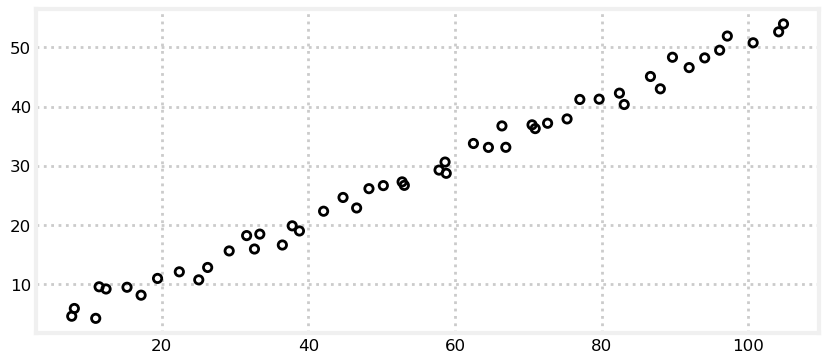That looks correct. The slope is 1/2, as we would expect with a velocity of (2, 1), and the data seems to start at near (6, 4). It doesn't look realistic. This is still a 'textbook' representation. As we continue we will add complications that adds real world behavior.

### Choose the State Variables¶

As always, the first step is to choose our state variables. We are tracking in two dimensions and have a sensor that gives us a reading in each of those two dimensions, so we know that we have the two observed variables $x$ and $y$. If we created our Kalman filter using only those two variables the performance would not be very good because we would be ignoring the information velocity can provide to us. We will want to incorporate velocity into our equations as well. I will represent this as

$$\mathbf x = \begin{bmatrix}x & \dot x & y & \dot y\end{bmatrix}^\mathsf T$$

There is nothing special about this organization. I could have used $\begin{bmatrix}x & y & \dot x & \dot y\end{bmatrix}^\mathsf T$ or something less logical. I just need to be consistent in the rest of the matrices. I like keeping positions and velocities next to each other because it keeps the covariances between positions and velocities in the same sub block of the covariance matrix. In my formulation P[1,0] contains the covariance of of $x$ and $\dot x$. In the alternative formulation that covariance is at P[2, 0]. This gets worse as the number of dimension increases.

Let's pause and address how you identify the hidden variables. This example is somewhat obvious because we've already worked through the 1D case, but other problems won't be obvious There is no easy answer to this question. The first thing to ask yourself is what is the interpretation of the first and second derivatives of the data from the sensors. We do that because obtaining the first and second derivatives is mathematically trivial if you are reading from the sensors using a fixed time step. The first derivative is just the difference between two successive readings. In our tracking case the first derivative has an obvious physical interpretation: the difference between two successive positions is velocity.

Beyond this you can start looking at how you might combine the data from two or more different sensors to produce more information. This opens up the field of sensor fusion, and we will be covering examples of this in later sections. For now, recognize that choosing the appropriate state variables is paramount to getting the best possible performance from your filter. Once you have chosen hidden variables, you must run many tests to ensure that you are generating real results for them. The Kalman filter runs whatever model you give it; if your model cannot generate good information for the hidden variables the Kalman filter output will be nonsensical.

### Design State Transition Function¶

Our next step is to design the state transition function. Recall that the state transition function is implemented as a matrix $\mathbf F$ that we multiply with the previous state of our system to get the next state, like so.

$$\mathbf{\bar x} = \mathbf{Fx}$$

I will not belabor this as it is very similar to the 1-D case we did in the previous chapter. The state transition equations are

\begin{aligned} x &= 1x + \Delta t \dot x + 0y + 0 \dot y \\ v_x &= 0x + 1\dot x + 0y + 0 \dot y \\ y &= 0x + 0\dot x + 1y + \Delta t \dot y \\ v_y &= 0x + 0\dot x + 0y + 1 \dot y \end{aligned}

Laying it out that way shows us both the values and row-column organization required for $\small\mathbf F$. We convert this to matrix-vector form:

$$\begin{bmatrix}x \\ \dot x \\ y \\ \dot y\end{bmatrix} = \begin{bmatrix}1& \Delta t& 0& 0\\0& 1& 0& 0\\0& 0& 1& \Delta t\\ 0& 0& 0& 1\end{bmatrix}\begin{bmatrix}x \\ \dot x \\ y \\ \dot y\end{bmatrix}$$

So, let's do this in Python. It is very simple; the only thing new here is setting dim_z to 2. We will see why it is set to 2 in step 4.

In :
from filterpy.kalman import KalmanFilter

tracker = KalmanFilter(dim_x=4, dim_z=2)
dt = 1.   # time step 1 second

tracker.F = np.array([[1, dt, 0,  0],
[0,  1, 0,  0],
[0,  0, 1, dt],
[0,  0, 0,  1]])


### Design the Process Noise Matrix¶

FilterPy can compute the $\mathbf Q$ matrix for us. For simplicity I will assume the noise is a discrete time Wiener process - that it is constant for each time period. This assumption allows me to use a variance to specify how much I think the model changes between steps. Revisit the Kalman Filter Math chapter if this is not clear.

In :
from scipy.linalg import block_diag
from filterpy.common import Q_discrete_white_noise

q = Q_discrete_white_noise(dim=2, dt=dt, var=0.001)
tracker.Q = block_diag(q, q)
print(tracker.Q)

[[0.    0.001 0.    0.   ]
[0.001 0.001 0.    0.   ]
[0.    0.    0.    0.001]
[0.    0.    0.001 0.001]]


Here I assume the noise in x and y are independent, so the covariances between any x and y variable should be zero. This allows me to compute $\mathbf Q$ for one dimension, and then use block_diag to copy it for the x and y axis.

### Design the Control Function¶

We haven't yet added controls to our robot, so there is nothing to be done for this step. The KalmanFilter class initializes B to zero under the assumption that there is no control input, so there is no code to write. If you like, you can be explicit and set tracker.B to 0, but as you can see it already has that value.

In :
tracker.B


### Design the Measurement Function¶

The measurement function $\mathbf H$ defines how we go from the state variables to the measurements using the equation $\mathbf z = \mathbf{Hx}$. In this case we have measurements for (x,y), so we will design $\mathbf z$ as $\begin{bmatrix}x & y\end{bmatrix}^\mathsf T$ which is dimension 2x1. Our state variable is size 4x1. We can deduce the required size for $\textbf{H}$ by recalling that multiplying a matrix of size MxN by NxP yields a matrix of size MxP. Thus,

$$(2\times 1) = (a\times b)(4 \times 1) = (2\times 4)(4\times 1)$$

So, $\textbf{H}$ is 2x4.

Filling in the values for $\textbf{H}$ is easy because the measurement is the position of the robot, which is the $x$ and $y$ variables of the state $\textbf{x}$. Let's make this slightly more interesting by deciding we want to change units. The measurements are returned in feet, and that we desire to work in meters. $\textbf{H}$ changes from state to measurement, so the conversion is $\mathsf{feet} = \mathsf{meters} / 0.3048$. This yields

$$\mathbf H = \begin{bmatrix} \frac{1}{0.3048} & 0 & 0 & 0 \\ 0 & 0 & \frac{1}{0.3048} & 0 \end{bmatrix}$$

which corresponds to these linear equations

\begin{aligned} z_x &= (\frac{x}{0.3048}) + (0* v_x) + (0*y) + (0 * v_y) = \frac{x}{0.3048}\\ z_y &= (0*x) + (0* v_x) + (\frac{y}{0.3048}) + (0 * v_y) = \frac{y}{0.3048} \end{aligned}

This is a simple problem, and we could have found the equations directly without going through the dimensional analysis that I did above. But it is useful to remember that the equations of the Kalman filter imply a specific dimensionality for all of the matrices, and when I start to get lost as to how to design something it is useful to look at the matrix dimensions.

Here is my implementation:

In :
tracker.H = np.array([[1/0.3048, 0, 0,        0],
[0,        0, 1/0.3048, 0]])


### Design the Measurement Noise Matrix¶

We assume that the $x$ and $y$ variables are independent white Gaussian processes. That is, the noise in x is not in any way dependent on the noise in y, and the noise is normally distributed about the mean 0. For now let's set the variance for $x$ and $y$ to be 5 meters$^2$. They are independent, so there is no covariance, and our off diagonals will be 0. This gives us:

$$\mathbf R = \begin{bmatrix}\sigma_x^2 & \sigma_y\sigma_x \\ \sigma_x\sigma_y & \sigma_{y}^2\end{bmatrix} = \begin{bmatrix}5&0\\0&5\end{bmatrix}$$

It is a $2{\times}2$ matrix because we have 2 sensor inputs, and covariance matrices are always of size $n{\times}n$ for $n$ variables. In Python we write:

In :
tracker.R = np.array([[5., 0],
[0, 5]])
tracker.R

Out:
array([[5., 0.],
[0., 5.]])

### Initial Conditions¶

For our simple problem we will set the initial position at (0,0) with a velocity of (0,0). Since that is a pure guess, we will set the covariance matrix $\small\mathbf P$ to a large value.

$$\mathbf x = \begin{bmatrix}0\\0\\0\\0\end{bmatrix}, \, \mathbf P = \begin{bmatrix}500&0&0&0\\0&500&0&0\\0&0&500&0\\0&0&0&500\end{bmatrix}$$

The Python implementation is

In :
tracker.x = np.array([[0, 0, 0, 0]]).T
tracker.P = np.eye(4) * 500.


### Implement the Filter¶

Design is complete, now we just have to write the code to run the filter and output the data in the format of our choice. We will run the code for 30 iterations.

In :
from filterpy.stats import plot_covariance_ellipse
from kf_book.book_plots import plot_filter

R_std = 0.35
Q_std = 0.04

def tracker1():
tracker = KalmanFilter(dim_x=4, dim_z=2)
dt = 1.0   # time step

tracker.F = np.array([[1, dt, 0,  0],
[0,  1, 0,  0],
[0,  0, 1, dt],
[0,  0, 0,  1]])
tracker.u = 0.
tracker.H = np.array([[1/0.3048, 0, 0, 0],
[0, 0, 1/0.3048, 0]])

tracker.R = np.eye(2) * R_std**2
q = Q_discrete_white_noise(dim=2, dt=dt, var=Q_std**2)
tracker.Q = block_diag(q, q)
tracker.x = np.array([[0, 0, 0, 0]]).T
tracker.P = np.eye(4) * 500.
return tracker

# simulate robot movement
N = 30
sensor = PosSensor((0, 0), (2, .2), noise_std=R_std)

zs = np.array([sensor.read() for _ in range(N)])

# run filter
robot_tracker = tracker1()
mu, cov, _, _ = robot_tracker.batch_filter(zs)

for x, P in zip(mu, cov):
# covariance of x and y
cov = np.array([[P[0, 0], P[2, 0]],
[P[0, 2], P[2, 2]]])
mean = (x[0, 0], x[2, 0])
plot_covariance_ellipse(mean, cov=cov, fc='g', std=3, alpha=0.5)

#plot results
zs *= .3048 # convert to meters
plot_filter(mu[:, 0], mu[:, 2])
plot_measurements(zs[:, 0], zs[:, 1])
plt.legend(loc=2)
plt.xlim(0, 20);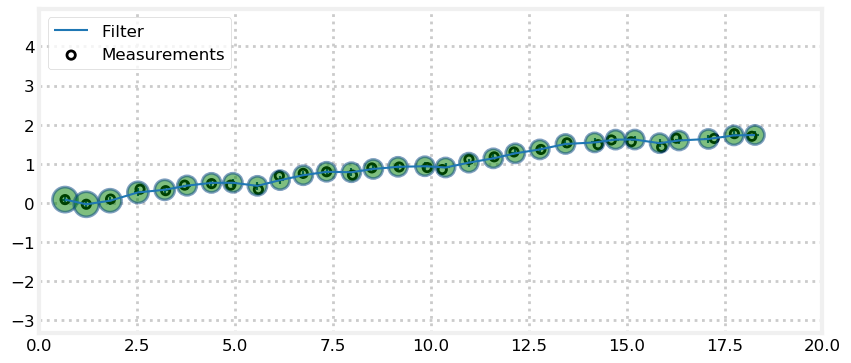I encourage you to play with this, setting $\mathbf Q$ and $\mathbf R$ to various values. However, we did a fair amount of that sort of thing in the last chapters, and we have a lot of material to cover, so I will move on to more complicated cases where we will also have a chance to experience changing these values.

I plotted the $3\sigma$ the covariance ellipse for $x$ and $y$ in green. Can you explain their shape? Perhaps you were expecting a tilted ellipse, as in the last chapters. If so, recall that in those chapters we were not plotting $x$ against $y$, but $x$ against $\dot x$. $x$ is correlated to $\dot x$, but $x$ is not correlated or dependent on $y$. Therefore our ellipses are not tilted. Furthermore, the noise for both $x$ and $y$ are modeled to have the same noise standard deviation. If we were to set R to, for example,

$$\mathbf R = \begin{bmatrix}1&0\\0&.5\end{bmatrix}$$

we would be telling the Kalman filter that there is more noise in $x$ than $y$, and our ellipses would be longer than they are tall.

The final value for $\mathbf P$ tells us everything we need to know about the correlation between the state variables. If we look at the diagonal alone we see the variance for each variable. In other words $\mathbf P_{0,0}$ is the variance for x, $\mathbf P_{1,1}$ is the variance for $\dot x$, $\mathbf P_{2,2}$ is the variance for y, and $\mathbf P_{3,3}$ is the variance for $\dot y$. We can extract the diagonal of a matrix using numpy.diag().

In :
print(np.diag(robot_tracker.P))

[0.007 0.003 0.007 0.003]


The covariance matrix contains four $2{\times}2$ matrices that you should be able to easily pick out. This is due to the correlation of $x$ to $\dot x$, and of $y$ to $\dot y$. The upper left hand side shows the covariance of $x$ to $\dot x$.

In :
c = robot_tracker.P[0:2, 0:2]
print(c)
plot_covariance_ellipse((0, 0), cov=c, fc='g', alpha=0.2)

[[0.007 0.003]
[0.003 0.003]]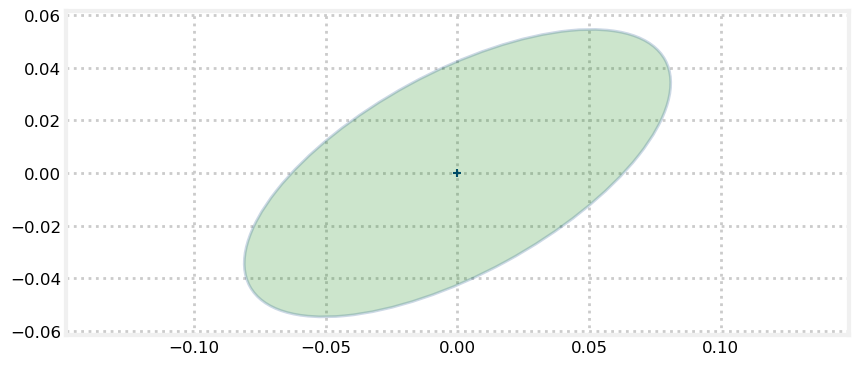The covariance contains the data for $x$ and $\dot x$ in the upper left because of how it is organized. Recall that entries $\mathbf P_{i,j}$ and $\mathbf P_{j,i}$ contain $\sigma_i\sigma_j$.

Finally, let's look at the lower left side of $\mathbf P$, which is all 0s. Why 0s? Consider $\mathbf P_{3,0}$. That stores the term $\sigma_3\sigma_0$, which is the covariance between $\dot y$ and $x$. These are independent, so the term will be 0. The rest of the terms are for similarly independent variables.

In :
robot_tracker.P[2:4, 0:2]

Out:
array([[0., 0.],
[0., 0.]])

## Filter Order¶

We have only studied tracking position and velocity. It has worked well, but only because I have been selecting problems for which this is an appropriate choice. You now have enough experience with the Kalman filter to consider this in more general terms.

What do I mean by order? In the context of these system models it is the number of derivatives required to accurately model a system. Consider a system that does not change, such as the height of a building. There is no change, so there is no need for a derivative, and the order of the system is zero. We could express this in an equation as $x = 312.5$.

A first order system has a first derivative. For example, change of position is velocity, and we can write this as

$$v = \frac{dx}{dt}$$

which we integrate into the Newtonian equation

$$x = vt + x_0.$$

This is also called a constant velocity model, because of the assumption of a constant velocity.

A second order system has a second derivative. The second derivative of position is acceleration, with the equation

$$a = \frac{d^2x}{dt^2}$$

which we integrate into

$$x = \frac{1}{2}at^2 +v_0t + x_0.$$

This is also known as a constant acceleration model.

Another, equivalent way of looking at this is to consider the order of the polynomial. The constant acceleration model has a second derivative, so it is second order. Likewise, the polynomial $x = \frac{1}{2}at^2 +v_0t + x_0$ is second order.

When we design the state variables and process model we must choose the order of the system we want to model. Let's say we are tracking something with a constant velocity. No real world process is perfect, and so there will be slight variations in the velocity over short time period. You might reason that the best approach is to use a second order filter, allowing the acceleration term to deal with the slight variations in velocity.

In practice that doesn't work well. To thoroughly understand this issue let's see the effects of using a process model that does not match the order of the system being filtered.

First we need a system to filter. I'll write a class to simulate an object with constant velocity. Essentially no physical system has a truly constant velocity, so on each update we alter the velocity by a small amount. I also write a sensor to simulate Gaussian noise in a sensor. The code is below, and I plot an example run to verify that it is working correctly.

In :
from kf_book.book_plots import plot_track

class ConstantVelocityObject(object):
def __init__(self, x0=0, vel=1., noise_scale=0.06):
self.x = x0
self.vel = vel
self.noise_scale = noise_scale

def update(self):
self.vel += randn() * self.noise_scale
self.x += self.vel
return (self.x, self.vel)

def sense(x, noise_scale=1.):
return x + randn()*noise_scale

np.random.seed(124)
obj = ConstantVelocityObject()

xs, zs = [], []
for i in range(50):
x = obj.update()
z = sense(x)
xs.append(x)
zs.append(z)

xs = np.asarray(xs)

plot_track(xs[:, 0])
plot_measurements(range(len(zs)), zs)
plt.legend(loc='best');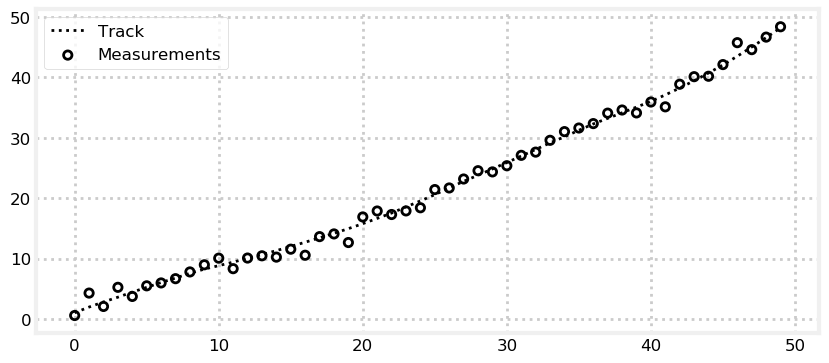I am satisfied with this plot. The track is not perfectly straight due to the noise that we added to the system - this could be the track of a person walking down the street, or perhaps of an aircraft being buffeted by variable winds. There is no intentional acceleration here, so we call it a constant velocity system. Again, you may be asking yourself that since there is in fact a tiny bit of acceleration going on why would we not use a second order Kalman filter to account for those changes? Let's find out.

How does one design a zero order, first order, or second order Kalman filter? We have been doing it all along, but just not using those terms. It might be slightly tedious, but I will elaborate fully on each - if the concept is clear to you feel free to skim a bit.

### Zero Order Kalman Filter¶

A zero order Kalman filter is just a filter that tracks with no derivatives. We are tracking position, so that means we only have a state variable for position (no velocity or acceleration), and the state transition function also only accounts for position. Using the matrix formulation we would say that the state variable is

$$\mathbf x = \begin{bmatrix}x\end{bmatrix}$$

The state transition function is very simple. There is no change in position, so we need to model $x=x$; in other words, x at time t+1 is the same as it was at time t. In matrix form, our state transition function is

$$\mathbf F = \begin{bmatrix}1\end{bmatrix}$$

The measurement function is very easy. Recall that we need to define how to convert the state variable $\mathbf x$ into a measurement. We will assume that our measurements are positions. The state variable only contains a position, so we get

$$\mathbf H = \begin{bmatrix}1\end{bmatrix}$$

Let's write a function that constructs and returns a zero order Kalman filter.

In :
def ZeroOrderKF(R, Q, P=20):
""" Create zero order Kalman filter.
Specify R and Q as floats."""

kf = KalmanFilter(dim_x=1, dim_z=1)
kf.x = np.array([0.])
kf.R *= R
kf.Q *= Q
kf.P *= P
kf.F = np.eye(1)
kf.H = np.eye(1)
return kf


### First Order Kalman Filter¶

A first order Kalman filter tracks a first order system, such as position and velocity. We already did this for the dog tracking problem above, so this should be very clear. But let's do it again.

A first order system has position and velocity, so the state variable needs both of these. The matrix formulation could be

$$\mathbf x = \begin{bmatrix}x\\\dot x\end{bmatrix}$$

So now we have to design our state transition. The Newtonian equations for a time step are:

\begin{aligned} x_t &= x_{t-1} + v\Delta t \\ v_t &= v_{t-1}\end{aligned}

Recall that we need to convert this into the linear equation

$$\begin{bmatrix}x\\\dot x\end{bmatrix} = \mathbf F\begin{bmatrix}x\\\dot x\end{bmatrix}$$

Setting

$$\mathbf F = \begin{bmatrix}1 &\Delta t\\ 0 & 1\end{bmatrix}$$

gives us the equations above.

Finally, we design the measurement function. The measurement function needs to implement

$$\mathbf z = \mathbf{Hx}$$

Our sensor still only reads position, so it should take the position from the state, and 0 out the velocity and acceleration, like so:

$$\mathbf H = \begin{bmatrix}1 & 0 \end{bmatrix}$$

This function constructs and returns a first order Kalman filter.

In :
def FirstOrderKF(R, Q, dt):
""" Create first order Kalman filter.
Specify R and Q as floats."""

kf = KalmanFilter(dim_x=2, dim_z=1)
kf.x = np.zeros(2)
kf.P *= np.array([[100, 0], [0, 1]])
kf.R *= R
kf.Q = Q_discrete_white_noise(2, dt, Q)
kf.F = np.array([[1., dt],
[0., 1]])
kf.H = np.array([[1., 0]])
return kf


### Second Order Kalman Filter¶

A second order Kalman filter tracks a second order system, such as position, velocity and acceleration. The state variable will be

$$\mathbf x = \begin{bmatrix}x\\\dot x\\\ddot{x}\end{bmatrix}$$

So now we have to design our state transition. The Newtonian equations for a time step are:

\begin{aligned} x_t &= x_{t-1} + v_{t-1}\Delta t + 0.5a_{t-1} \Delta t^2 \\ v_t &= v_{t-1} + a_{t-1}\Delta t \\ a_t &= a_{t-1}\end{aligned}

Recall that we need to convert this into the linear equation

$$\begin{bmatrix}x\\\dot x\\\ddot{x}\end{bmatrix} = \mathbf F\begin{bmatrix}x\\\dot x\\\ddot{x}\end{bmatrix}$$

Setting

$$\mathbf F = \begin{bmatrix}1 & \Delta t &.5\Delta t^2\\ 0 & 1 & \Delta t \\ 0 & 0 & 1\end{bmatrix}$$

gives us the equations above.

Finally, we design the measurement function. The measurement function needs to implement

$$z = \mathbf{Hx}$$

Our sensor still only reads position, so it should take the position from the state, and 0 out the velocity, like so:

$$\mathbf H = \begin{bmatrix}1 & 0 & 0\end{bmatrix}$$

This function constructs and returns a second order Kalman filter.

In :
def SecondOrderKF(R_std, Q, dt, P=100):
""" Create second order Kalman filter.
Specify R and Q as floats."""

kf = KalmanFilter(dim_x=3, dim_z=1)
kf.x = np.zeros(3)
kf.P[0, 0] = P
kf.P[1, 1] = 1
kf.P[2, 2] = 1
kf.R *= R_std**2
kf.Q = Q_discrete_white_noise(3, dt, Q)
kf.F = np.array([[1., dt, .5*dt*dt],
[0., 1.,       dt],
[0., 0.,       1.]])
kf.H = np.array([[1., 0., 0.]])
return kf


## Evaluating Filter Order¶

Now we can run each Kalman filter against the simulation and evaluate the results.

How do we evaluate the results? We can do this qualitatively by plotting the track and the Kalman filter output and eyeballing the results. However, a rigorous approach uses mathematics. Recall that the system covariance matrix $\mathbf P$ contains the computed variance and covariances for each of the state variables. The diagonal contains the variance. Remember that roughly 99% of all measurements fall within $3\sigma$ if the noise is Gaussian. If this is not clear please review the Gaussian chapter before continuing, as this is an important point.

So we can evaluate the filter by looking at the residuals between the estimated state and actual state and comparing them to the standard deviations which we derive from $\mathbf P$. If the filter is performing correctly 99% of the residuals will fall within $3\sigma$. This is true for all the state variables, not just for the position.

I must mention that this is only true for simulated systems. Real sensors are not perfectly Gaussian, and you may need to expand your criteria to, say, $5\sigma$ with real sensor data.

So let's run the first order Kalman filter against our first order system and access its performance. You can probably guess that it will do well, but let's look at it using the standard deviations.

First, let's write a routine to generate the noisy measurements for us.

In :
def simulate_system(Q, count):
obj = ConstantVelocityObject(x0=.0, vel=0.5, noise_scale=Q)
xs, zs = [], []
for i in range(count):
x = obj.update()
z = sense(x)
xs.append(x)
zs.append(z)
return np.array(xs), np.array(zs)


And now a routine to perform the filtering and save the output in a Saver object.

In :
from filterpy.common import Saver

def filter_data(kf, zs):
s = Saver(kf)
kf.batch_filter(zs, saver=s)
s.to_array()
return s


Now we are prepared to run the filter and look at the results.

In :
from kf_book.book_plots import plot_kf_output

R, Q = 1, 0.03
xs, zs = simulate_system(Q=Q, count=50)

kf = FirstOrderKF(R, Q, dt=1)
data1 = filter_data(kf, zs)

plot_kf_output(xs, data1.x, data1.z)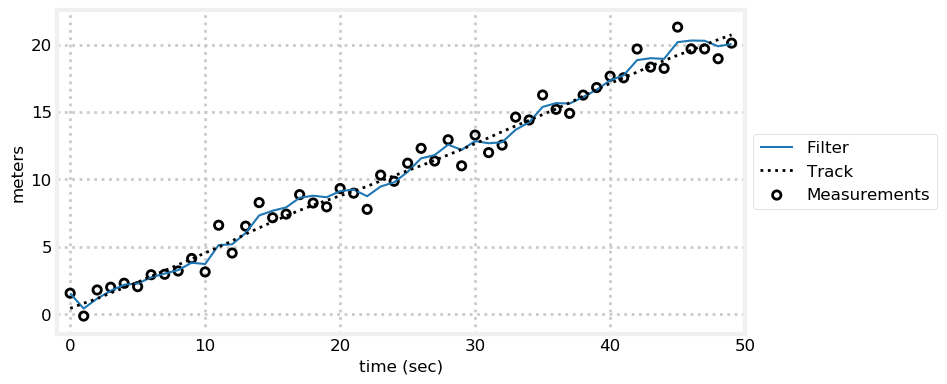It looks like the filter is performing well, but it is hard to tell exactly how well. Let's look at the residuals and see if they help. We'll do this a lot, so I'll write a function to plot them.

In :
from kf_book.book_plots import plot_residual_limits, set_labels

def plot_residuals(xs, data, col, title, y_label, stds=1):
res = xs - data.x[:, col]
plt.plot(res)
plot_residual_limits(data.P[:, col, col], stds)
set_labels(title, 'time (sec)', y_label)

In :
plot_residuals(xs[:, 0], data1, 0,
title='First Order Position Residuals(1$\sigma$)',
y_label='meters')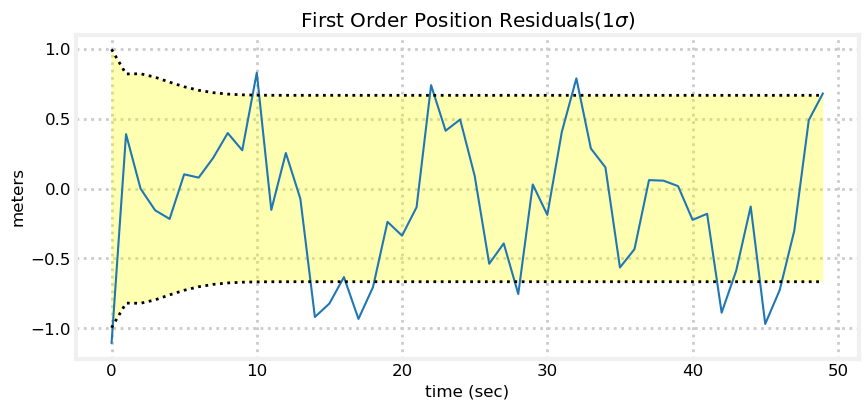How do we interpret this plot? The residual is drawn as the jagged line - the difference between the measurement and the predicted position. If there was no measurement noise and the Kalman filter prediction was always perfect the residual would always be zero. So the ideal output would be a horizontal line at 0. We can see that the residual is centered around 0, so this gives us confidence that the noise is Gaussian (because the errors fall equally above and below 0). The yellow area between dotted lines show the theoretical performance of the filter for 1 standard deviations. In other words, approximately 68% of the errors should fall within the dotted lines. The residual falls within this range, so we see that the filter is performing well, and that it is not diverging.

Let's look at the residuals for velocity.

In :
plot_residuals(xs[:, 1], data1, 1,
title='First Order Velocity Residuals(1$\sigma$)',
y_label='meters/sec')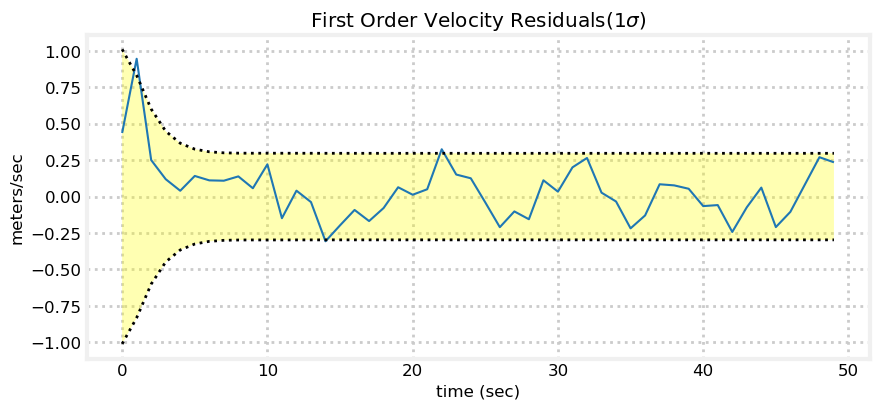Again, as expected, the residual falls within the theoretical performance of the filter, so we feel confident that the filter is well designed for this system.

Now let's do the same thing using the zero order Kalman filter. All of the code and math is largely the same, so let's just look at the results without discussing the implementation much.

In :
kf0 = ZeroOrderKF(R, Q)
data0 = filter_data(kf0, zs)
plot_kf_output(xs, data0.x, data0.z)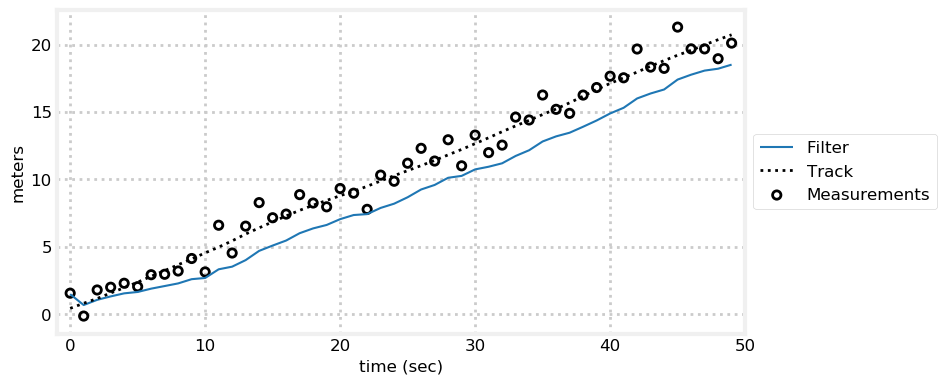As we would expect, the filter has problems. Think back to the g-h filter, where we incorporated acceleration into the system. The g-h filter always lagged the input because there were not enough terms to allow the filter to adjust quickly enough to the changes in velocity. On every predict() step the Kalman filter assumes that there is no change in position - if the current position is 4.3 it will predict that the position at the next time period is 4.3. Of course, the actual position is closer to 5.3. The measurement, with noise, might be 5.4, so the filter chooses an estimate part way between 4.3 and 5.4, causing it to lag the actual value of 5.3 by a significant amount. This same thing happens in the next step, the next one, and so on. The filter never catches up.

This raises a very important point. The assumption of 'constant' is an assumption of constant-ness between discrete samples only. The filter's output can still change over time.

Now let's look at the residuals. We are not tracking velocity, so we can only look at the residual for position.

In :
plot_residuals(xs[:, 0], data0, 0,
title='Zero Order Position Residuals(3$\sigma$)',
y_label='meters',
stds=3)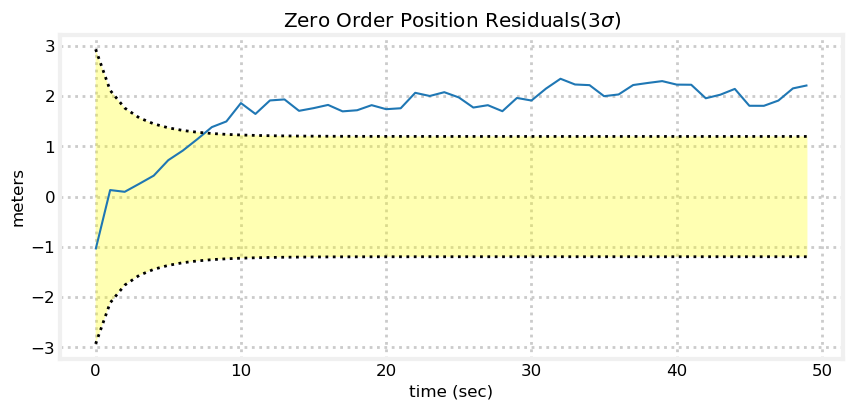We can see that the filter diverges almost immediately. After a few seconds the residual exceeds the bounds of three standard deviations. It is important to understand that the covariance matrix $\mathbf P$ is only reporting the theoretical performance of the filter assuming all of the inputs are correct. In other words, this Kalman filter is diverging, but $\mathbf P$ implies that the Kalman filter's estimates are getting better and better with time because the variance is getting smaller. The filter has no way to know that you are lying to it about the system. This is sometimes referred to a smug filter - it is overconfident in its performance.

In this system the divergence is immediate and striking. In many systems it will only be gradual, and/or slight. It is important to look at charts like these for your systems to ensure that the performance of the filter is within the bounds of its theoretical performance.

Now let's try a second order system. This might strike you as a good thing to do. After all, we know there is a bit of noise in the movement of the simulated object, which implies there is some acceleration. Why not model the acceleration with a second order model? If there is no acceleration, the acceleration should just be estimated to be 0, right?. But is that what happens? Think about it before going on.

In :
kf2 = SecondOrderKF(R, Q, dt=1)
data2 = filter_data(kf2, zs)
plot_kf_output(xs, data2.x, data2.z)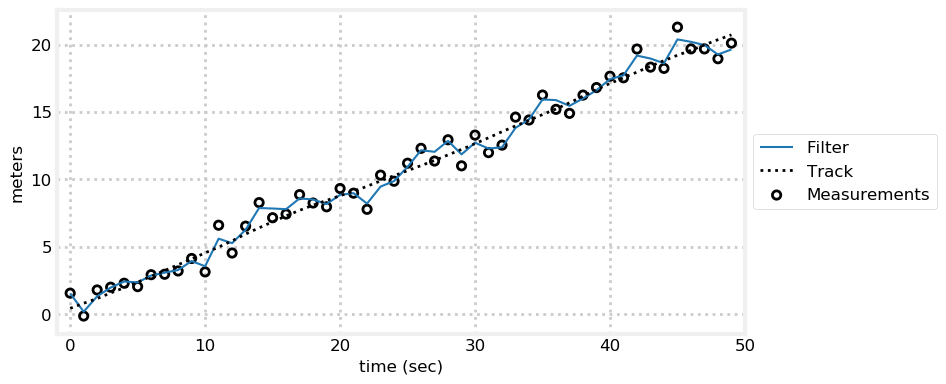Did this perform as you expected?

We can see that second order filter performs poorly compared to the first order filter. Why? This filter models acceleration, and so the large changes in the measurement gets interpreted as acceleration instead of noise. Thus the filter closely tracks the noise. Not only that, but it overshoots the noise in places if the noise is consistently above or below the track because the filter incorrectly assumes an acceleration that does not exist, and so it's prediction goes further and further away from the track on each measurement. This is not a good state of affairs.

Still, the track doesn't look horrible. Let's see the story that the residuals tell. I will add a wrinkle here. The residuals for the second order system do not look terrible in that they do not diverge or exceed three standard deviations. However, it is very telling to look at the residuals for the first order vs the second order filter, so I have plotted both on the same graph.

In :
res2 = xs[:, 0] - data2.x[:, 0]
res1 = xs[:, 0] - data1.x[:, 0]

plt.plot(res1, ls="--", label='order 1')
plt.plot(res2, label='order 2')
plot_residual_limits(data2.P[:, 0, 0])
set_labels('Second Order Position Residuals',
'meters', 'time (sec)')
plt.legend();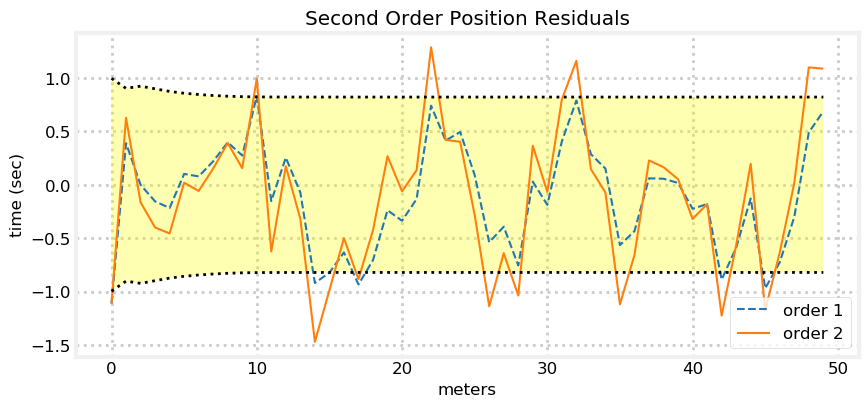The second order position residuals are slightly worse than the residuals of the first order filter, but they still fall within the theoretical limits of the filter. There is nothing very alarming here.

Now let's look at the residuals for the velocity.

In :
res2 = xs[:, 1] - data2.x[:, 1]
res1 = xs[:, 1] - data1.x[:, 1]

plt.plot(res2, label='order 2')
plt.plot(res1, ls='--', label='order 1')
plot_residual_limits(data2.P[:, 1, 1])
set_labels('Second Order Velocity Residuals',
'meters/sec', 'time (sec)')
plt.legend();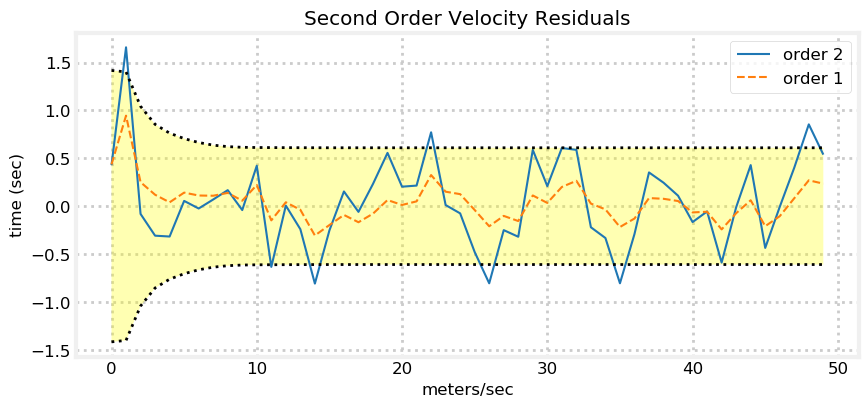Here the story is very different. While the residuals of the second order system fall within the theoretical bounds of the filter's performance, we can see that the residuals are far worse than for the first order filter. This is the usual result for this scenario. The filter is assuming that there is acceleration that does not exist. It mistakes noise in the measurement as acceleration and this gets added into the velocity estimate on every predict cycle. Of course the acceleration is not actually there and so the residual for the velocity is much larger than its optimum.

I have one more trick up my sleeve. We have a first order system; i.e. the velocity is more-or-less constant. Real world systems are never perfect, so of course the velocity is never exactly the same between time periods. When we use a first order filter we account for that slight variation in velocity with the process noise. The matrix $\mathbf Q$ is computed to account for this slight variation. If we move to a second order filter we are now accounting for the changes in velocity. Perhaps now we have no process noise, and we can set $\mathbf Q$ to zero!

In :
kf2 = SecondOrderKF(R, 0, dt=1)
data2 = filter_data(kf2, zs)
plot_kf_output(xs, data2.x, data2.z)